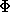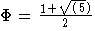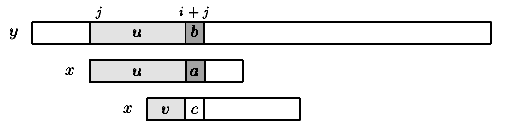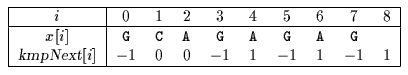Next: Simon algorithm Up: ESMAJ Prev: Morris-Pratt algorithm

# Knuth-Morris-Pratt algorithm

Main features
• performs the comparisons from left to right;
• preprocessing phase in O(m) space and time complexity;
• searching phase in O(n+m) time complexity (independent from the alphabet size);
• delay bounded by log(m) whereis the golden ratio ().
Description

The design of the Knuth-Morris-Pratt algorithm follows a tight analysis of the Morris and Pratt algorithm. Let us look more closely at the Morris-Pratt algorithm. It is possible to improve the length of the shifts.

Consider an attempt at a left position j, that is when the the window is positioned on the text factor y[j .. j+m-1]. Assume that the first mismatch occurs between x[i] and y[i+j] with 0 < i < m. Then, x[0 .. i-1] = y[j .. i+j-1] =u and a = x[iy[i+j]=b.

When shifting, it is reasonable to expect that a prefix v of the pattern matches some suffix of the portion u of the text. Moreover, if we want to avoid another immediate mismatch, the character following the prefix v in the pattern must be different from a. The longest such prefix v is called the tagged border of u (it occurs at both ends of u followed by different characters in x).

This introduces the notation: let kmpNext[i] be the length of the longest border of x[0 .. i-1] followed by a character c different from x[i] and -1 if no such tagged border exits, for 0 < im. Then, after a shift, the comparisons can resume between characters x[kmpNext[i]] and y[i+j] without missing any occurrence of x in y, and avoiding a backtrack on the text (see figure 7.1). The value of kmpNext is set to -1.Figure 7.1: Shift in the Knuth-Morris-Pratt algorithm (v border of u and cb).

The table kmpNext can be computed in O(m) space and time before the searching phase, applying the same searching algorithm to the pattern itself, as if x=y.

The searching phase can be performed in O(m+n) time. The Knuth-Morris-Pratt algorithm performs at most 2n-1 text character comparisons during the searching phase. The delay (maximal number of comparisons for a single text character) is bounded by log(m) whereis the golden ratio ().

The C code
```void preKmp(char *x, int m, int kmpNext[]) {
int i, j;

i = 0;
j = kmpNext = -1;
while (i < m) {
while (j > -1 && x[i] != x[j])
j = kmpNext[j];
i++;
j++;
if (x[i] == x[j])
kmpNext[i] = kmpNext[j];
else
kmpNext[i] = j;
}
}

void KMP(char *x, int m, char *y, int n) {
int i, j, kmpNext[XSIZE];

/* Preprocessing */
preKmp(x, m, kmpNext);

/* Searching */
i = j = 0;
while (j < n) {
while (i > -1 && x[i] != y[j])
i = kmpNext[i];
i++;
j++;
if (i >= m) {
OUTPUT(j - i);
i = kmpNext[i];
}
}
}

```
The example

Preprocessing phaseThe kmpNext table

Searching phase

References
• AHO, A.V., 1990, Algorithms for finding patterns in strings. in Handbook of Theoretical Computer Science, Volume A, Algorithms and complexity, J. van Leeuwen ed., Chapter 5, pp 255-300, Elsevier, Amsterdam.
• AOE, J.-I., 1994, Computer algorithms: string pattern matching strategies, IEEE Computer Society Press.
• BAASE, S., VAN GELDER, A., 1999, Computer Algorithms: Introduction to Design and Analysis, 3rd Edition, Chapter 11, pp. ??-??, Addison-Wesley Publishing Company.
• BAEZA-YATES R., NAVARRO G., RIBEIRO-NETO B., 1999, Indexing and Searching, in Modern Information Retrieval, Chapter 8, pp 191-228, Addison-Wesley.
• BEAUQUIER, D., BERSTEL, J., CHRÉTIENNE, P., 1992, Éléments d'algorithmique, Chapter 10, pp 337-377, Masson, Paris.
• CORMEN, T.H., LEISERSON, C.E., RIVEST, R.L., 1990. Introduction to Algorithms, Chapter 34, pp 853-885, MIT Press.
• CROCHEMORE, M., 1997. Off-line serial exact string searching, in Pattern Matching Algorithms, ed. A. Apostolico and Z. Galil, Chapter 1, pp 1-53, Oxford University Press.
• CROCHEMORE, M., HANCART, C., 1999, Pattern Matching in Strings, in Algorithms and Theory of Computation Handbook, M.J. Atallah ed., Chapter 11, pp 11-1--11-28, CRC Press Inc., Boca Raton, FL.
• CROCHEMORE, M., LECROQ, T., 1996, Pattern matching and text compression algorithms, in CRC Computer Science and Engineering Handbook, A. Tucker ed., Chapter 8, pp 162-202, CRC Press Inc., Boca Raton, FL.
• CROCHEMORE, M., RYTTER, W., 1994, Text Algorithms, Oxford University Press.
• GONNET, G.H., BAEZA-YATES, R.A., 1991. Handbook of Algorithms and Data Structures in Pascal and C, 2nd Edition, Chapter 7, pp. 251-288, Addison-Wesley Publishing Company.
• GOODRICH, M.T., TAMASSIA, R., 1998, Data Structures and Algorithms in JAVA, Chapter 11, pp 441-467, John Wiley & Sons.
• GUSFIELD, D., 1997, Algorithms on strings, trees, and sequences: Computer Science and Computational Biology, Cambridge University Press.
• HANCART, C., 1992, Une analyse en moyenne de l'algorithme de Morris et Pratt et de ses raffinements, in Théorie des Automates et Applications, Actes des 2e Journées Franco-Belges, D. Krob ed., Rouen, France, 1991, PUR 176, Rouen, France, 99-110.
• HANCART, C., 1993. Analyse exacte et en moyenne d'algorithmes de recherche d'un motif dans un texte, Ph. D. Thesis, University Paris 7, France.
• KNUTH D.E., MORRIS (Jr) J.H., PRATT V.R., 1977, Fast pattern matching in strings, SIAM Journal on Computing 6(1):323-350.
• SEDGEWICK, R., 1988, Algorithms, Chapter 19, pp. 277-292, Addison-Wesley Publishing Company.
• SEDGEWICK, R., 1988, Algorithms in C, Chapter 19, Addison-Wesley Publishing Company.
• SEDGEWICK, R., FLAJOLET, P., 1996, An Introduction to the Analysis of Algorithms, Chapter ?, pp. ??-??, Addison-Wesley Publishing Company.
• STEPHEN, G.A., 1994, String Searching Algorithms, World Scientific.
• WATSON, B.W., 1995, Taxonomies and Toolkits of Regular Language Algorithms, Ph. D. Thesis, Eindhoven University of Technology, The Netherlands.
• WIRTH, N., 1986, Algorithms & Data Structures, Chapter 1, pp. 17-72, Prentice-Hall.Next: Simon algorithm Up: ESMAJ Prev: Morris-Pratt algorithm

e-mails: {Christian.Charras, Thierry.Lecroq }@laposte.net
Tue Jan 14 15:03:31 MET 1997